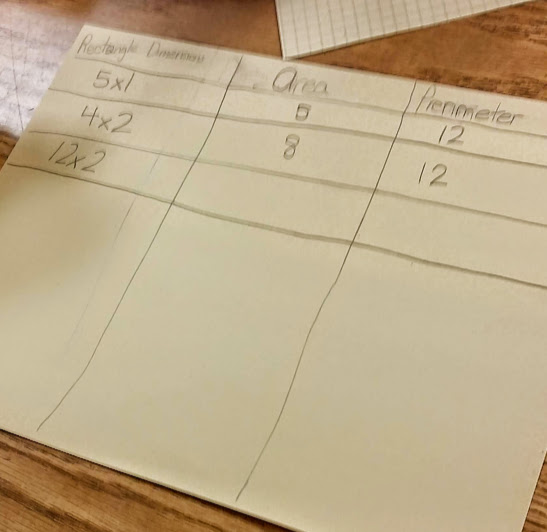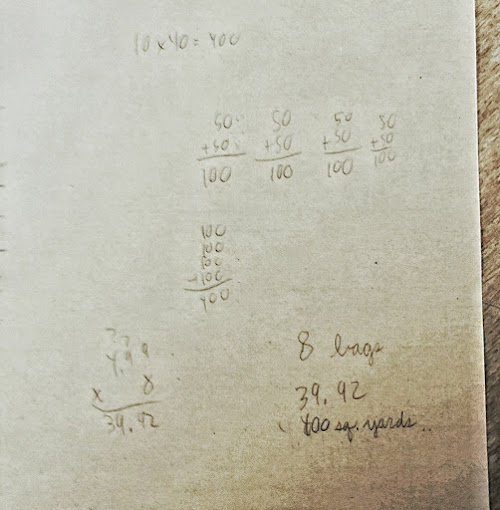# Patterns with Perimeter and Area

11 teachers like this lesson
Print Lesson

## Objective

SWBAT apply the area and perimeter formulas for rectangles in real world situations.

#### Big Idea

In this lesson, students construct rectangles to build conceptual understanding of area and perimeter and then work on a real life problem.

## Warm Up

5 minutes

I begin this lesson with this song. My students have heard this before and it's one of their favorites. They can't help but sing along. This song is an excellent way for my students to review area and perimeter and get ready for the concept development portion of this lesson. This is also an excellent way to differentiate instructions. Students who do not remember what area and perimeter are can sing along in a low-risk fun way.

## Concept Development

50 minutes

Students worked with area and perimeter in third grade and many can find the area and perimeter of given rectangles. Some students may even be able to state the formulas for finding the perimeter and area of a rectangle. However, many students get these formulas confused unless they have sufficient opportunity to use models as they construct their understanding.

I begin this lesson by instructing students to draw a rectangle with a perimeter measuring 12 units. (Students use the grid paper.) Then I have a student volunteer share their rectangle for all to see using the document camera. Students’ rectangles may be  of the following whole unit dimensions: 1 x 5, 2 x 4, or 3 x 3.

Next, I ask students to find the area of their rectangle and to record in a table.  I show students how to set up a three column chart to record their data.  See an example of a students chart below.Watch and look at this video.  A student is questioning another student about whether or not the rectangle he is showing has a perimeter of 12.  Notice the discourse and dialogue that is happening with these students.  You can also hear others in the room commenting as well. It is important to note that for many discussion and math activities in my classroom, I do not require students to raise their hands. In this video, you can see that if students were required to raise their hands, the discussion would have been much more stifled and not authentic.

Then I ask students if they notice anything about the relationship between the area and perimeter.  (I want students to respond with things like; perimeter is length plus width times two, while area is length times width. Some students will also notice that the closer the rectangle is to a square, or a square, the greater the area if the perimeters are the same)

Next, I direct students to draw and find the area of all the rectangles they can that have a perimeter of 24 units.

Rectangle dimensions will be: 1 x 11, 2 x 10, 3 x 9, 4 x 8, 5 x 7, and 6 x 6.  Students work on this for about 7 minutes.  Once most students are finished, I lead a discussion about patterns much like I did when students found rectangles with perimeters of 12 units.

As students work, I circulate around the room and assist as necessary.  In this video, you can hear and see a student struggling to find the area of a rectangle he has created. Listen to my questions and comments as I guide this student to realize he has an array so he can multiply.

For the remainder of the lesson, students solve the following problems independently-  area and perimeter

The community center has decided to move the parking area to the back of the building and replace the front with a grass lawn. The lawn is rectangular shaped with a length of 10 yards and a width of 40 yards. A bag of grass seed covers 50 square yards at a cost of \$4.99 per bag. What is the total area of the new lawn? How many bags of grass seed will they need to buy?  What is the total cost to renovate the front lawn with the new grass?

You can see a student's work on this problem below.Mr. Lehman keeps his chickens in a square pen with an area of 100 square feet. What is the length of one side of the chicken pen?

Mason's parents are re-carpeting his bedroom. The dimensions of the room are 17 x 12.  How many square feet of carpet do they need to buy for the entire room?